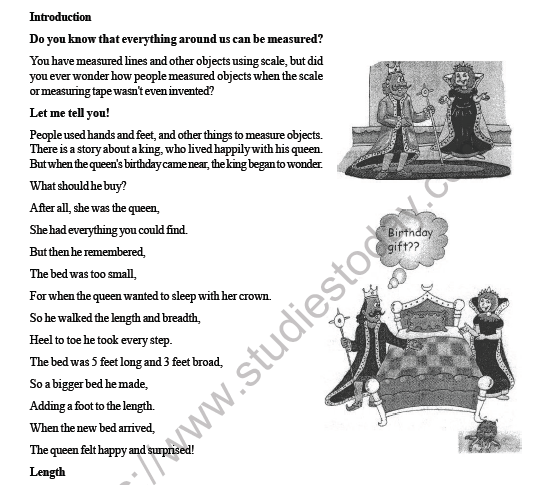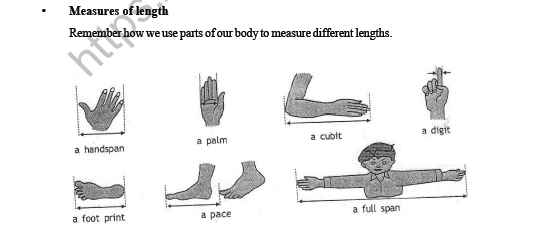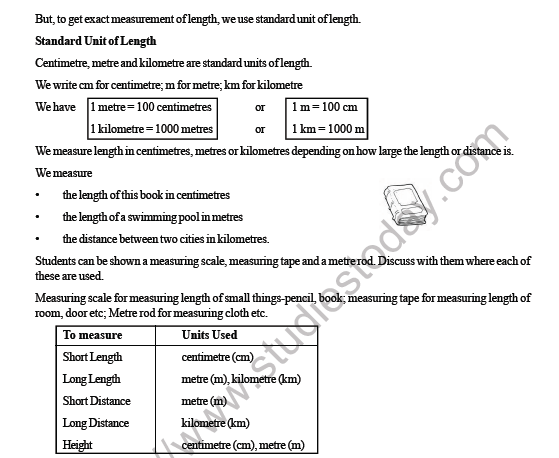# CBSE Class 3 Maths Measurement Worksheet

Download printable Mathematics Class 3 Worksheets in pdf format, CBSE Class 3 Maths Measurement Worksheet has been prepared as per the latest syllabus and exam pattern issued by CBSE, NCERT and KVS. Also download free pdf Mathematics Class 3 Assignments and practice them daily to get better marks in tests and exams for Grade 3. Free chapter wise worksheets with answers have been designed by Standard 3 teachers as per latest examination pattern

## Measurement Class 3 Mathematics Worksheet Pdf

Class 3 Mathematics students should refer to the following printable worksheet in Pdf for Measurement in Grade 3. This test paper with questions and solutions for Standard 3 Mathematics will be very useful for tests and exams and help you to score better marks

### Class 3 Mathematics Worksheet for MeasurementClick for more Mathematics Study Material
 CBSE Class 3 Mathematics Worksheet Set P CBSE Class 3 Maths Fun with Numbers Worksheet CBSE Class 3 Maths Geometry and Patterns Question Bank CBSE Class 3 Mathematics Worksheet Set J Class 3 Mathematics Printable Worksheet Set F CBSE Class 3 Maths Addition Division Worksheet CBSE Class 3 Maths Time Question Bank CBSE Class 3 Maths Rupees and Paise Worksheet CBSE Class 3 Maths Multiplication Summary and Worksheet CBSE Class 3 Mathematics Worksheet Set Q CBSE Class 3 Maths Give and Take Worksheet CBSE Class 3 Maths Geometry and Patterns Worksheet CBSE Class 3 Mathematics Worksheet Set K Class 3 Mathematics Printable Worksheet Set G CBSE Class 3 Maths Addition Question Bank CBSE Class 3 Maths Time Worksheet CBSE Class 3 Maths Shapes and Designs Worksheet CBSE Class 3 Maths Multiplication Worksheet Class 3 Mathematics Printable Worksheet Set A CBSE Class 3 Mathematics Worksheet Set R CBSE Class 3 Maths How Many Times Worksheet CBSE Class 3 Maths Measurement Question Bank CBSE Class 3 Mathematics Worksheet Set L Class 3 Mathematics Printable Worksheet Set H CBSE Class 3 Maths Addition Worksheet CBSE Class 3 Maths Smart Charts Worksheet CBSE Class 3 Maths Number upto 9999 Question Bank CBSE Class 3 Mathematics Worksheet Set S Class 3 Mathematics Printable Worksheet Set B CBSE Class 3 Maths Jugs and Mugs Worksheet CBSE Class 3 Maths Money Question Bank CBSE Class 3 Mathematics Worksheet Set M Class 3 Mathematics Printable Worksheet Set I CBSE Class 3 Maths Division Question Bank CBSE Class 3 Maths Time Goes On Worksheet CBSE Class 3 Maths Number upto 9999 Worksheet CBSE Class 3 Mathematics Worksheet Set T Class 3 Mathematics Printable Worksheet Set C CBSE Class 3 Maths Long and Short Worksheet CBSE Class 3 Maths Measurement Worksheet CBSE Class 3 Mathematics Worksheet Set N CBSE Class 3 Maths Can We Share Worksheet CBSE Class 3 Maths Fraction Question Bank CBSE Class 3 Maths Where to Look From Worksheet CBSE Class 3 Maths Substraction Question Bank CBSE Class 3 Mathematics Worksheet Set U Class 3 Mathematics Printable Worksheet Set D CBSE Class 3 Maths Numbers Worksheet CBSE Class 3 Maths Money Worksheet CBSE Class 3 Mathematics Worksheet Set O CBSE Class 3 Maths Fun With Give and Take Worksheet CBSE Class 3 Maths Fraction Worksheet CBSE Class 3 Maths Who is Heavier Worksheet CBSE Class 3 Mathematics Worksheet Set V CBSE Class 3 Maths Substraction Worksheet Class 3 Mathematics Printable Worksheet Set E CBSE Class 3 Maths Play with Patterns Worksheet CBSE Class 3 Maths Multiplication Question Bank

## Latest NCERT & CBSE News

Read the latest news and announcements from NCERT and CBSE below. Important updates relating to your studies which will help you to keep yourself updated with latest happenings in school level education. Keep yourself updated with all latest news and also read articles from teachers which will help you to improve your studies, increase motivation level and promote faster learning

### CBSE Class 10 Revised Syllabus

Last year CBSE had to reduce the syllabus because of the pandemic situation but it was not very effective because there were no examinations. This year to avoid any confusion and conflict, CBSE has decided to reduce the syllabus into term 1 and term 2. 50 percent of...

### Score well in Class 12 English Boards Exam

12th Board exams are an important part of students' lives. The marks obtained in the board exam decide the college in which one can study. In class 12 the syllabus of each and every subject increases vastly and it is difficult to cover up every point. In English also...

### How To Solve Unseen Passages In English

Unseen passages may contain one or many paragraphs. This is one of the important yet easy parts for a student to get marks. Students should thoroughly study and understand the passage to answer the related questions. The unseen passages are there just to test the...

### MCQ Question based CBSE examination

For 2021-22 CBSE has launched MCQ question-based examination for Term 1 & Term 2 board examinations. The entire syllabus has been divided into two parts each including 50% of the entire syllabus. To score well, students must practice as per the new CBSE term-wise...

×